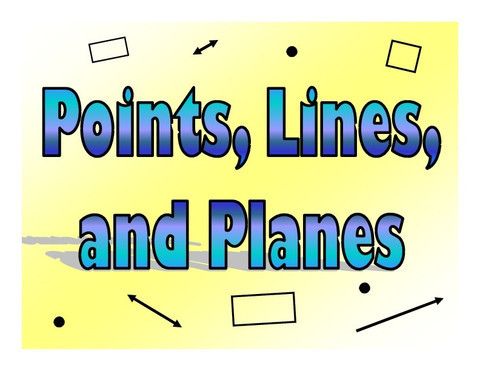Points, Lines & Planes
3 years ago
tales
Save
Edit
Host a game
Live GameLive
Homework
Solo Practice
Practice15 QuestionsShow answers
• Question 1
20 seconds
Q. A line is named by...
Any 2 points on the line, or a lowercase script letter.
Any 3 points on the line.
Any 1 point on the line.
Any 3 points on the line, or a uppercase script letter.
• Question 2
20 seconds
Q. A plane is named by...
Any 1 point on the plane.
Any 3 collinear points on the plane or a lowercase script letter.
Any 3 non-collinear points on the plane or an uppercase script letter.
All points on the plane that aren't part of a line.
• Question 3
20 seconds
Q. Two lines intersect at a ....
angle
point
line
intersection
• Question 4
20 seconds
Q. Two planes intersect at a...
line
point
plane
letter
• Question 5
30 secondsQ. Name 4 collinear points
HMNO
IKNO
MJOL
HNKI
• Question 6
30 secondsQ. A line that contains point M.
Line q
Line p
Line r
Line NH
• Question 7
30 secondsQ. An example of 2 non-collinear points.
D, E
C, B
M, E
F, B
• Question 8
30 secondsQ. Give another name for line k.
Line BC
Line BCE
Line ED
Line k is the only name, there isn't another name.
• Question 9
30 secondsQ. A plane containing point A.
Plane DEA
Plane k
Plane CDF
Plane F
• Question 10
30 secondsQ. Name 3 coplaner points.
ZRW
VZX
VWX
WYZ
• Question 11
30 secondsQ. How many planes appear in the figure?
4
1
6
5
• Question 12
30 secondsQ. How many planes contain point W?
3
2
4
1
• Question 13
30 secondsQ. Name the intersection of Plane R and plane ZVY
point Y
Line VY
Line ZV
Point V
• Question 14
30 secondsQ. Give another name for plane Q.
Plane FEGI
Plane FEG
Plane m
Plane FGI
• Question 15
30 secondsQ. Are points D and E collinear or coplanar?